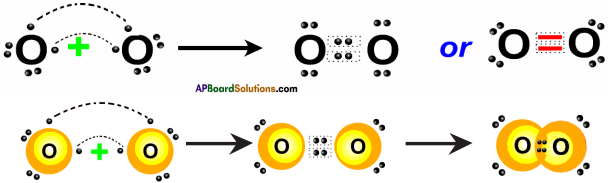# AP SSC 10th Class Chemistry Important Questions Chapter 10 Chemical Bonding

AP State Board Syllabus AP SSC 10th Class Physical Science Important Questions Chapter 10 Chemical Bonding

## AP State Syllabus SSC 10th Class Chemistry Important Questions 10th Chemical Bonding

### 10th Class Chemistry 10th Lesson Chemical Bonding 1 Mark Important Questions and Answers

Question 1.
What is a chemical bond? (AP March 2015)
An attractive force between two atoms in a molecule is called a chemical bond.

Question 2.
Write the names of any two compounds which have ionic bond. (TS June 2016)
1) NaCl
2) MgCl2

Question 3.
Draw the structural diagram of Ammonia molecule as per the valence-shell electron pair repulsion theory. (TS March 2016)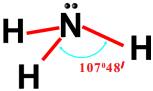Question 4.
Show the formation of HC/ molecule with Lewis dot structures using the information given below. (TS March 2017)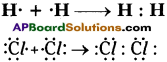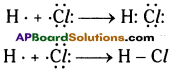Question 5.
Explain, why bonding angle (HOH) in water is 104° 31′ instead of 109° 28′? (TS June 2018)
Due to the repulsion between the lone pair of electrons and bond pair of electrons in water molecule the bond angle will be 104° 31′ rather than 109° 28′.

Question 6.
Imagine and write what type of ion can be formed generally by an atom of element with low ionisation energy, low electron affinity with high atomic size? (AP March 2019)
Cation or positivity charged ion can be formed generally by an atom of element with low ionisation energy, low electron affinity with high atomic size.Question 7.
Draw the structure of Methane molecule and mention bond angle. (TS March 2019)
Structure of methane CH4. Tetrahedral structure with bond angle 109°28′.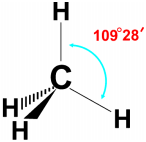Bond Angle is 109°28′

Question 8.
Why do elements form chemical bonds? (TS SCERT: 2019-20)

• Elements are unstable if they contain less than eight electrons (octet) in their valency shell.
• Hence, they form chemical bond with other elements to get octet in their valency shell.

Question 9.
How is covalent bond formed?
A covalent bond is formed by the sharing of electrons between two atoms.
Ex : Bonding in H2 molecule, O2 molecule, N2 molecule, etc.

Question 10.
What is ‘Octet rule’?
Octet rule :
Presence of 8 electrons in the outermost shell of an atom or a molecule is called ‘octet rule’.

Question 11.
What is ‘Bond length’?
Bond length :
It is the inner-nucleus distance between the two atoms in a molecule. It is measured in Angstrom, 1 Å = 10-8 cm.Question 12.
How is a cation formed?
A cation is formed when an atom loses electrons.

Question 13.
How is an anion formed?
An anion is formed when an atom gains electrons.

Question 14.
Which type of compounds are more soluble in polar solvents?
Ionic compounds are more soluble in polar solvents.

Question 15.
Which compounds exhibit high melting and boiling points?
Ionic compounds exhibit high melting and boiling points.

Question 16.
What is electronic configuration?
A systematic arrangement of electrons in the atomic orbits is called electronic configuration.Question 17.
Why are molecules more stable than atoms?
Molecules have lower energy than that of the combined atoms. Molecules are more stable than atoms since chemical species with lower energy are more stable.

Question 18.
When two oppositely charged ions are engaged in a bond, it is known as ionic linkage.

Question 19.
On which factors do anions depend?

1. Atomic size
2. Ionisation potential
3. Electron affinity
4. Electronegativity

Question 20.
How do you know the valence of a metal?
The number of electrons lost from a metal atom is the valence of its element which is equal to its group number.
Ex : Na and Mg have valence 1 and 2 respectively.

Question 21.
How do you know the valence of a non-metal?
The number of electrons gained by a non-metal element for its atom is its valency, which is equal to 8 – its group number. Ex : The valency of chlorine is (8 – 7) = 1.

Question 22.
What is Tonic bond’?
The electrostatic attractive force that keeps cation and anion together to form a new electrically neutral compounds is called ionic bond.Question 23.
Why do atoms combine and form molecules?
The energy of molecule is less than the total energy of constituent atoms. Therefore atoms combine and go to a stable state of lower energy.

Question 24.
What is ‘orbital concept of bond formation’?
Atoms with half-filled or vacant orbitals try to get paired electrons in those orbitals by bond formation, i.e. by losing, gaining or sharing of electrons.

Question 25.
Name the bonds present in the molecules
i) BaCl2
ii) C2H4.
i) In BaCl2 – Ionic.
ii) In C2H4 – Covalent (double bond H2C = CH2).

Question 26.
Why are ionic compounds good electrolytes?

• Electrolytes produce ions in solution, which carry current.
• Ionic compounds in the fused state and aqueous solutions contain ions moving freely. Hence they conduct electricity.

Question 27.
When is ionic bond formed between atoms?
Ionic bond is readily formed between atoms of elements with a low ionisation energy and atoms of elements with high electronegativity.

Question 28.
What is crystal lattice’?
In a crystal of an ionic compound, each ion is surrounded by oppositely charged ions. The ions arrange themselves at an optimum distance with regular periodicity in a well-defined three-dimensional network called crystal lattice.Question 29.
What is Lattice energy’?
The energy released when gaseous positive and negative ions are brought together from infinity to form one mole ionic crystals is called lattice energy.

Question 30.
How is a σ (sigma) bond formed?
By the axial or head on overlap of pure orbitals or hybrid orbitals of two atoms.

Question 31.
How is a π (pi) bond formed?
By the lateral or parallel overlap of only pure atomic orbitals after the a bond formation.

Question 32.
Which type of atoms easily enter ionic bonding?
An atom with low ionization potential and another with high electron affinity.

Question 33.
What is a polar bond?
A covalent bond in a heteroatomic molecule.
Eg : HCl.

Question 34.
What is meant by inter-nuclear axis?
The hypothetical line joining the centre of nuclei of two atoms in a molecule.

Question 35.
What are multiple bonds?
Double and triple bonds are multiple bonds.Question 36.
How many a and π bonds are in O2 molecule?
One σ and one π bond.

Question 37.
What are Lewis structures?
The symbol of the atom gives the core (or kernel) of the atom, in which valence electrons are shown as dots (•), circles (O) or crosses (x). Thus in Na, Na represents the core of sodium atom and the cross (x) represents the valence electron of sodium.

Question 38.
What are the structures of sodium chloride and calcium chloride crystals?
Sodium chloride has face centered cubic structure. Calcium chloride has body centered cubic structure.

Question 39.
NaCl dissolves in water but not in benzene. Explain.
NaCl dissolves in water because of hydration. Water being a polar molecule has positive and negative ends which hydrate Na+ and Cl ions. Benzene being non-polar cannot solvate the ions of NaCl.

Question 40.
What are the bond angles in H2O and NH3 molecule?
Bond angle in H2O molecule is 104° 30′.
Bond angle in NH3 molecule is 107°.

Question 41.
What are the forces present in an ionic bond?
Electrostatic forces of attraction are present in ionic bonds.

Question 42.
Which forces are weaker forces and where are they operative?

1. Van der Waal’s forces are very weak forces.
2. They are operative between non-polar molecules.

Question 43.
Which compounds exhibit low melting and boiling points?
Covalent compounds exhibit low melting and boiling points when compared to ionic compounds.

Question 44.
How are cations formed?
The metal atoms lose electrons to form positively charged ions or cations.Question 45.
Why is a molecule of hydrogen more stable than the uncombined atoms?
When a molecule of hydrogen is formed from the atoms, energy is released (104 Kcal/mol). Thus the molecules possessing lower energy are more stable than the atoms.

Question 46.
How many sigma and pi bonds are present in acetylene molecule between carbon atoms?
$$\mathrm{HC} \equiv \mathrm{CH}$$, one sigma and two pi bonds are present.

Question 49.
In case of ionic substances, a more appropriate term is formula weight, rather than ‘molecular weight’. Why?
Molecules are not present in ionic substances. Only ions are present. The formula of an ionic substance represents the simpler ratio of ions in one mole of crystal. Hence a more appropriate term is ‘formula weight’.

Question 50.
Write a short note on bond angles.
It is the average angle between two adjacent atoms bonded to the central atom in a molecule. Molecules with larger bond angles are more stable than those with smaller angles.

Question 51.
Sulphur dioxide is a diamagnetic molecule. Explain.
Sulphur dioxide (SO2) is a diamagnetic molecule because it has all electrons paired (no free electrons).

Question 52.
What is an ion?
An ion is an electrically charged atom (or group of atoms).

Question 53.
What is a Coordination number?
The number of ions of opposite charge that surround a given ion of given charge is known as coordination number of that given ion. –

Question 54.
What factors affect or which factors influence the formation cation or anion?
Factors affecting formation of anion or cation are :

1. Atomic size,
2. Ionization potential,
3. Electron affinity,
4. Electronegativity.

Question 55.
Two elements X and Y have the following configurations.
X = 1s² 2s² 2p6 3s² 3p6 4s²
Y = 1s² 2s² 2p6 3s² 3p5
What is the formula of the compound?
The electronic configuration of X is 1s² 2s² 2p6 3s² 3p6 4s².
So its valency is 2.
The electronic configuration of Y is 1s² 2s² 2p6 3s² 3p5.
So its valency is 1.
∴ The formula of the compound is XY2.Question 56.
The electronegativities of two elements are 1 and 3. What type of bond is formed between the two elements? Why?
The electronegativity difference of elements = 3 – 1 = 2.
So the electronegativity difference is more than 1.9. Therefore the bond formed between the elements is ionic in nature.

Question 57.
What do you mean by Doublet configuration?
If two electrons are present in valence shell, then it is called doublet configuration.
Eg.: Helium exhibits doublet configuration.

Question 58.
Write Lewis symbol of potassium and calcium.
Potassium – $$\dot{\mathrm{K}}$$ and Calcium – •Ca•

Question 59.
Which of the following is / are true?
1) Ionic compounds exist as solids.
2) Ionic compounds have high melting and boiling points.
3) Ionic compounds conduct electricity in solid state.
4) Ionic compounds have low melting and boiling points.
Ionic compounds generally exists as solids and they have very high melting and boiling points and they conduct electricity in aqueous or molten state.
So first and second statements are true.

Question 60.
How many a and n bonds are there in acetylene molecule?
The structure of acetylene is given by
$$\mathrm{H}-\mathrm{C} \equiv-\mathrm{H}$$
So they are 3a and 2n bonds.

Question 61.
What is the valency of carbon in CO2?
The valency of oxygen is 2. So the valency of carbon = 2 × 2 = 4.

Question 62.
Which of the following does not get Helium configuration during formation of chemical bonding?
1) Hydrogen,
2) Lithium,
3) Beryllium,
4) Oxygen.
Oxygen attains Neon configuration when it undergoes chemical bondings and the rest will attain Helium configuration.

Question 63.
Write the valencies of following elements.
a) Be
b) N and what is the compound formed when these two reacted?
a) The electronic configuration of Be is 1s² 2s². So its valency is 2.
b) The electronic configuration of Nitrogen is 1s² 2s² 2p³. So its valency is 3.
∴ Formula of the compound is Be3N2.

Question 64.
Which of the following solutions are conductors of electricity?
a) Sugar solution,
b) Alcohol solution,
c) Glucose solution,
d) Salt solution.
Salt solution is good conductor of electricity because common salt (NaCl) is an ionic compound and the rest are covalent compounds.Question 65.
Why are noble gases (inert gases) stable?

1. Noble gases are stable because their outermost orbit contains 8 or 2 electrons.
2. Noble gases exist an individual atoms.

### 10th Class Chemistry 10th Lesson Chemical Bonding 2 Marks Important Questions and Answers

Question 1.
Represent each of the following molecules using Lewis notation. (TS March 2015)
(i) Calcium and Chlorine to form Calcium chloride.
(ii) Formation of Oxygen molecule from Oxygen atoms.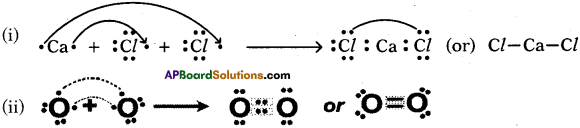Question 2.
Between a neutral atom and its cations which has bigger size? Why? (TS June 2016)

• A neutral atom has bigger in size than its cation.
• A cation has more protons and nucleus of the cation attracts the electrons in the outermost orbital more.
• Hence, the radius of the anion decreases. It means size of the anion decreases.
• So, a neutral atom is bigger than its cation.

Question 3.
Draw the diagram to show the formation of Oxygen molecule by Valence bond theory. (TS March 2017)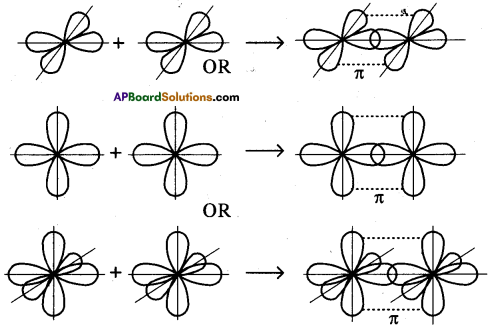Question 4.
Explain Ionic bond with suitable example. (TS June 2018)
1) Sodium (Na) looses one electron and forms Sodium ion (Na+).
Na → Na+ + 1e

2) Chlorine (Cl) gains one electron and forms chloride ion (Cl).
Cl + 1e → Cl

3) Positive sodium ion (Na+) and negative chloride (Ct) ion come together due to electrostatic forces, participates in ionic bond and form NaCl.
Na+ + Cl → NaClQuestion 5.
Give two examples of each to ionic and covalent compounds. (AP SCERT: 2019-20)
1) Ionic compounds eg : NaCl, MgCl2
2) Covalent compounds eg : Cl2, HCl

Question 6.
Distinguish between a sigma and a pi bond.

 Sigma bond Pi bond 1. It is formed by the end-end on overlap of orbitals. 1. It is formed by the lateral overlap of orbitals. 2. It has independent existence. 2. It has no independent existence. 3. It is a strong bond. Because axial overlap is more. 3. It is a weak bond. Because lateral overlap is less. 4. There can be only one a bond. 4. There can be one or two 7t bonds between two atoms. 5. All orbitals form ‘o’ bond. 5. Only p, d orbitals form a bond.

Question 7.

• Hydrogen bond is formed between molecules in which hydrogen atom is attached to an atom of an element with large electronegativity and very small size (F, O, N). Because in hydrogen bond the molecules associate themselves and hence possess higher B.P’s and M.P’s.
• The hydrogen bond formed between two molecules is called inter-molecular hydrogen bond.
• The hydrogen bond formed between different groups of the same molecule is called intra-molecular hydrogen bond.

Question 8.
Bring out the difference between ionic and covalent bonds.

 Ionic bond Covalent bond 1. It is formed by transference of electrons from one atom to the other. 1. It is formed by the sharing of electron pairs by two atoms. 2. Electrostatic. 2. Not electrostatic, but rigid. 3. Ionic substances are formed by ionic bonds. 3. Molecules are formed by covalent bonds. 4. Non-directional. 4. Directional.

Question 9.
What is hybridisation?
In the formation of molecules, the atomic orbitals of the atoms may hybridise.

1. It is the process of mixing up of atomic orbitals of an atom to form identical hybrid orbitals.
2. This takes place only during the formation of bond.
3. There should not be much difference in the energies of the orbitals that hybridise.
4. The number of hybrid orbitals formed is equal to the number of hybridising atomic orbitals.
5. Hybrid orbitals form a bonds only not n bonds.

Question 10.
What is ionisation? Give one example.
1) The process of removal of electron (s) from an atom or molecule is termed as ionisation.
Eg : Na → Na+ + e

2) Dissociation of an ionic solid into constituent ions upon its dissolution in a suitable solvent, is also called ionisation.
Eg : NaCl(aq) → Na+(aq) + Cl(aq)

Question 11.
Write a short note on octet rule with example.
Atoms try to get 8 electrons in their outermost shell (inert gas structure) by combining with other atoms. In order to get 8 outer electrons, atoms may lose to, gain from or share electrons with other atoms.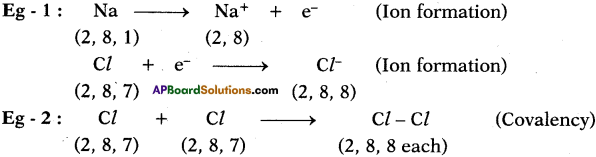Question 12.
What are the important characteristic features of hybridisation?

• Orbitals on a single atom only would undergo hybridization.
• The orbitals involved in hybridisation should not differ largely in their energies.
• The number of hybrid orbitals formed is equal to the number of hybridising orbitals.
• The hybrid orbitals form stronger directional bonds than the pure s, p, d atomic orbitals.
• It is the orbitals that undergo hybridisation and not the electrons.
• Concept of hybridisation is useful in explaining the shape of molecules.Question 13.
Why is a large amount of energy needed to remove an electron from a neutral gaseous neon atom than the energy needed to remove an electron from gaseous sodium atom?
Na (g) +118.4 K.cal → Na+ + e
Ne (g) + 497.0 K.cal → Ne+ + e

Orbitals which are fully-filled are very stable, so need large amount of energy to remove an electron from them.

Question 14.
What are the drawbacks of electronic theory of valence?
1) When covalent bond formed between any two atoms, irrespective of the nature of the atoms, the bond lengths and bond energies’ are expected to be same. But practically it was observed that bond lengths and bond energies are not same when the atoms that form the bond are different.

2) This theory fails to explain the shapes of molecules.

Question 15.
What is the structure of NaCl and write its coordination number of its constituent ions?
The structure of NaCl is face centred cubic lattice.
Its coordination number is 6 for Na+ and Cl.

Question 16.
Why is there absorption of energy in certain chemical reactions and release of energy in other reactions?
If bond dissociation energy of reactants is more than bond energy of products, then energy is absorbed in the chemical reaction.

If bond dissociation energy of reactants is less than bond energy of products, then energy is released in the chemical reaction.

Question 17.
Why do ionic compounds dissolve in polar solution and covalent compounds dissolve in non-polar solution?
Ionic compounds are polar in nature so they are soluble in polar solvents whereas covalent compounds are non-polar in nature so they are soluble in non-polar solvents.

Question 18.
Identify the following whether they are either oxidation or reduction reactions.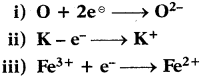i) Oxygen atom is gaining electrons. So it is reduction reaction.
ii) Potassium atom is losing electron. So it is oxidation reaction.
iii) Ferric ion is gaining electron. So it is reduction reaction.

Question 19.
Elements A, B, and C have atomic numbers 9, 20, and 10 respectively.
a) State which one is (i) a metal, (ii) a non-metal, (iii) chemically inert.
b) Write down the formula of the compound formed by two of the above elements.
a) i) Element with atomic number 20 is a metal because its electronic configuration is 2, 8, 8, 2. It belongs to 2nd group and the element is calcium.
ii) Element with atomic number 9 is a non-metal because its electronic configuration is 2, 7. So it belongs to 17th group and it is Fluorine.
iii) Element with atomic number 10 is inert gas because its electronic configuration is 2, 8 and the element is Neon.

b) The formula of compound formed between elements Calcium and Fluorine is CaF2.Question 20.
In the formation of the compound XY2, an atom X gives one electron to each Y atom. What is the nature of bond in XY2? Give two properties of XY2.
Two electrons are transferred from X to Y. X forms positive ion and Y forms a negative ion. So the bond formed is ionic in nature.
Properties of ionic compounds :
a) They are hard crystals.
b) They have high boiling point and melting point.
c) They are soluble in water.

Question 21.
Element X is a metal with valency 2. Element Y is a non-metal with valency 3.
i) Write equations to show X and Y form ions.
X is a metal with valency 2.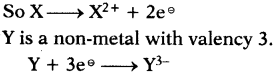ii) If Y is a di-atomic gas, write the equation for the direct combination of X and Y to form a compound.
4X + 3Y2 → 2X2Y3

Question 22.
What are the differences between sodium atom and sodium ion?

• Sodium atom is neutral whereas sodium ion has unipositive charge.
• The size of sodium ion is smaller than sodium atom.
• The properties of sodium ion is different when compared with sodium.

Question 23.
What is the percent of p-character in sp, sp² and sp³ hybrid orbitals?
In sp the p-character is 50%.
In sp² the p-character is 66.66%.
In sp³ the p-character is 75%.

Question 24.
What is the percent of s-character in sp, sp² and sp³ hybrid orbitals?
In sp hybrid orbitals s-character is 50%.
In sp² hybrid orbitals s-character is 33.33%.
In sp³ hybrid orbitals s-character is 25%.

Question 25.
A chemical compound has following Lewis notation.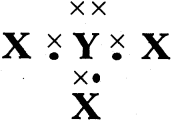a) How many valence electrons does element Y have?
b) What is the valency of element Y?
c) What is the valency of element X?
d) How many covalent bonds are there in the molecule?
e) Suggest a name for the element X and Y.
a) The valence electrons in Y are 5.
b) The valency of Y is 3.
c) The valency of X is 1.
d) There are three covalent bonds.
e) The element Y is Nitrogen and X is Hydrogen.

Question 26.
The electronic configurations of three elements.
X is 2, 6; Y is 2, 8, 7; Z is 2, 8,1.
In each ease given below state whether the bonding is ionic or covalent and give the formula of molecules of the compound formed,
a) Between two atoms of X.
As we move from left to right in a period non-metallic character increases. So Z is a metal and remaining two are non-metals.
Therefore between two atoms of X, there would be covalent bond. The formula of compound is X2.

b) Between the atom X and atom Z.
X is a non-metal and Z is a metal. So between these two atoms, there would be an ionic bond.
The valency of X is 2 and the valency of Z is 1.
So the formula of compound is Z2X.

c) Between the atom Y and atom Z.
Y is also non-metal and we know Z is metal.
The valency of Y is 1.
So the compound formed is ZY.Question 27.
Electronic configuration of X is 2, 8, 1 and electronic configuration of Y is 2, 8, 7. Explain what type of bond is formed between them.
The element with electronic configuration 2, 8,1 is sodium which is a metal. Similarly the element with electronic configuration 2, 8, 7 is chlorine which is a non-metal. The electronegativity difference between these two elements is more than 1.9 so they form ionic bond.

Question 28.
Complete the following statements.
i) The bond which is formed by transfer of an electron from one atom A to another atom B is called ……………
Ionic bond

ii) The atoms A and B become ions. Thus A – 2e = …………….. and B + e = ………….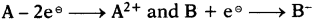iii) The formula of the compound is
The valency of A is 2 and valency of B is 1. So the compound formed is AB2.

Question 29.
i) What is the valency of Nitrogen in Ammonia?
The formula of Ammonia is NH3. We know valency of hydrogen is 1. So valency of Nitrogen is 3.

ii) What is the valency of Oxygen in Water?
The formula of water is H2O. The valency of hydrogen is 1. So the valency of oxygen is 2.

iii) What is the valency of Carbon in Carbon tetra chloride?
The formula of carbon tetra chloride is CCl4. The valency of chlorine is 1. So the valency of carbon is 4.

### 10th Class Chemistry 10th Lesson Chemical Bonding 4 Marks Important Questions and Answers

Question 1.
What is Hybridisation? Explain the formation of BeF, molecule using hybridisation. (AP June 2017)
Hybridisation :
Hybridisation is a phenomenon of intermixing of atomic orbitals of almost equal energy which are present in the outer shells of the atom and their reshuffling or redistribution into the same number of orbitals but with equal properties like energy and shape.

Formation of BeF2 molecule :

1. 4Be has electronic configuration 1s² 2s².
2. In excited state electronic configuration of 4Be is 1s² 2s¹ 2px¹
3. 4Be allows its 2s orbitals and 2px orbitals which contain unpaired electrons to intermix and redistribute to two identical orbitals.
4. The hybridisation is called ‘sp’ hybridisation and form two ‘sp’ hybrid orbitals. Each orbital contains one electron.
5. 9F has electronic configuration 1s² 2s² 2p5 (or) 1s² 2s² 2px² 2py² 2pz¹
6. F contains one unpaired electron.
7. Two Fluorine atoms come closure to Be and form two covalent bonds.
σsp – p and σsp – p
8. Now the BeF2 has (F $$\widehat{\mathrm{Be}}$$ F) angle of 180°.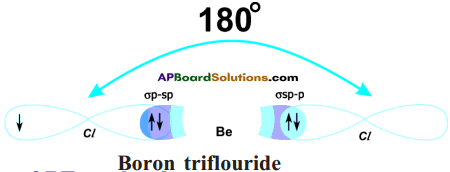Question 2.
The arrangement of electrons in different shells of atoms of 18th Group elements is given in the table.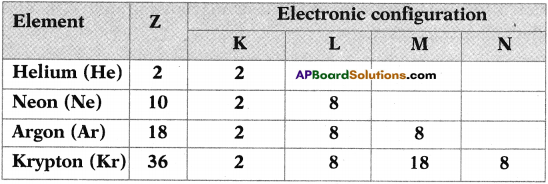Answer the following : (AP March 2017)
(i) What is the general electronic configuration of the above elements except He?
(ii) What is the valency of Argon?
(iii) Write Lewis dot structure of Neon.
(iv) Why the above elements do not take part in bond formation?
(i) ns² np6
(ii) Zero
(iii)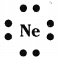(iv) They are stable as they have 8 electrons (except Helium) in their outer most orbit.

Question 3.
“Nitrogen and Hydrogen react to form a molecule of Ammonia (NH3). Carbon and Hydrogen react to form a molecule of Methane (CH4).”
For each reaction :
a) What is the valency of each of the atom involved in the reaction?
b) Draw the dot structure of the products that are formed. (AP June 2018)
a) In ammonia : Nitrogen valency – 3, Hydrogen valency – 1
In methane : Carbon valency – 4, Hydrogen valency – 1

b)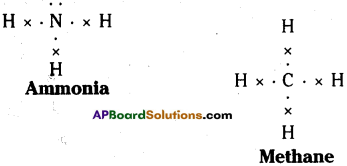Question 4.
Explain the formation of Boron tri-fluoride molecule by Hybridization. (TS June 2018)
1) Electron configuration of Boran at ground state.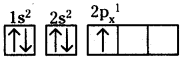2) Electron configuration of Boran at excited state is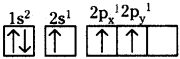3) As it forms three identical B-F bonds in BF3.
4) In excited state of Boran, one ‘s’ orbital and two ‘p’ orbitals undergoes hybridization and forms three sp² orbitals.
1s + 2p → 3sp²
5) Three sp² orbitals make end – on – end overlap with three ‘p’ orbitals of fluorine atoms.
6) They form three sp² – p sigma bonds.
7) In this way Boran tri-fluoride molecule is formed.
8)

Question 5.
Explain the formation of BF3 molecule with the help of Valence Bond theory? (TS March 2018)
Formation of BF3 molecule :

1. 5B has electronic configuration 1s² 2s² 2px.
2. The excited electronic configuration of 5B is 1s² 2s¹ 2px¹ 2py¹.
3. As it forms three identical B-F bonds in BF3
4. It is suggested that excited ‘B’ atom undergoes hybridisation.
5. There is an intermixing of 2s, 2px, 2py orbitals and their redistribution into three identical orbitals called sp² hybrid orbitals.
6. For three sp² orbitals to get separated to have minimum repulsion the angle between any two orbitals is 120° at the central atom and each sp² orbital gets one electron.
7. Now three fluorine atoms overlap their 2pz orbitals containing unpaired electrons (F9 1s² 2s² 2px² 2py² 2pz²) the three sp² orbitals of ‘B’ that contain unpaired electrons to form three ssp²-p bonds.

Question 6.
Explain the formation of N2 molecule using Valence Bond theory. (TS June 2019)
Formation of N, molecule (Valence Bond theory) :

1. 7N has electronic configuration 1s² 2s² 2px¹ 2py¹ 2pz.
2. Suppose that px orbital of one ‘N’ atom overlaps the ‘px‘ orbital of the other ‘N’ atom giving σ px – px bond along the inter – nuclear axis.
3. The py and pz orbitals of one ‘N’ atom overlap the py and pz orbital of other ‘N’ atom laterally, respectively perpendicular to inter – nuclear axis giving π py – py and π pz-pz bonds.
4. Therefore, N2 molecule has a triple bond between two nitrogen atoms.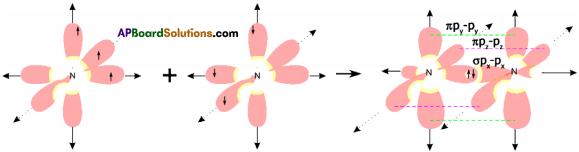Question 7.
If the electronic configurations of atoms A and B are 1s², 2s², 2p6, 3s², 3p¹ and 1s², 2s², 2p4 respectively, then
a) which atom forms negative ion?
Given electronic configuration of atom A is 1s² 2s² 2p6 3s² 3p¹, i.e. Aluminium and B is 1s² 2s² 2p4, i.e. Oxygen.

The atom ‘B’ tends to form negative ion by gaining two electrons in order to get nearest inert gas. Neon’s configuration is 1s² 2s² 2p6. So its valency is 2.

b) which atom forms positive ion?
The atom ‘A’ tends to form positive ion by losing three electrons in order to get nearest inert gas. Neon’s configuration is 1s² 2s² 2p6.

c) what is the valency of atom A?
Valency of atom ‘A’ is ‘3’.

d) what is the molecular formula of the compound formed by atoms A and B ?
According to Criss-Cross method, the molecular formula of the compound formed by atoms both A and B is A2B3, i.e. Al2O3.Question 8.
Writs the salient features of VSEPRT.
(OR)
Explain VSEPR theory.
The full form of VSEPRT is Valence Shell Electron Pair Repulsion Theory.

1. VSEPRT considers electrons in the valence shells which are in covalent bonds and in lone pairs as charge clouds that repel one another and stay as far apart as possible which will give specific shapes to molecules.
2. The presence of lone pair in the central atom causes slight distortion of bond angles from expected regular shape.
3. If the angle between lone pair and bond pair increases at the central atom due to more repulsion, the actual bond angles between atoms must be decreased.
4. If two bond pairs are present without any lone pair, the shape of the molecule is linear with bond angle 180°.
5. If three bond pairs are present without any lone pair, the shape of the molecule is trigonal planar with bond angle 120°.
6. If there are four bond pairs in the valency shell of central atom without lone pair, the shape is tetrahedron and the bond angle is 109°28′.
7. If there are three bond pairs and one lone pair, the shape of the molecule is pyramidal.
8. If there are two bond pairs and two lone pairs, the shape is V.

Question 9.
Do you think that a pair of Na+Cl as units would be present in the solid crystal? Explain.

• No, the electrostatic forces are non-directional.
• Therefore, it is not possible for one Na+ to be attracted by one Cl and vice-versa.
• Depending upon the size and charge of particular ion, number of oppositely charged ions get attracted by it, but, in a definite number.
• In sodium chloride crystal each Na+ is surrounded by 6 Cl and each Cl by six Na+ ions.
• Ionic compounds in the crystalline state consists of orderly arranged cations and anions held together by electrostatic forces of attractions in three dimensions.

Question 10.
Explain the valence bond theory.
Valence bond theory :

1. A covalent bond between two atoms is formed when the two atoms approach each other closely and one atom overlaps its valence orbital containing unpaired electron with the valence orbital of other atom that contains the unpaired electron of opposite spin.
2. The greater the overlapping of the orbitals, the stronger will be bond.
3. Valence bond theory gives a directional character to the bond when other than ‘s’ orbitals are involved.
4. Electron pair in the overlapping orbitals is shared bv both the atoms involved in the overlapping.
5. If orbitals overlap along inter-nucleus axis, they form a strong bond called sigma (σ) bond.
6. If the orbitals overlap laterally, they form a weak bond called pi (π) bond.Question 11.
a) Name the charged particles which attract one another to form ionic or electro-valent compounds.
The charged particles are ions which are formed due to transfer of electrons from atom of one element to atom of other element.

b) In the formation of ionic compounds, electrons are transferred from one element to another. How are electrons involved in the formation of covalent compounds?
In the formation of covalent bond electrons are mutually shared between atoms of elements.

c) The electronic configuration of Nitrogen is 2, 5. How many electrons in the outer- shell of a nitrogen atom are not involved in the formation of Nitrogen molecule?
In the formation of Nitrogen molecules three pairs of electrons are mutually shared by the two nitrogen atoms. So each Nitrogen has two electrons which do not take part in bonding. Those two electrons are called lone pair.

d) In the formation of magnesium chloride, name the substance that is oxidized and the substance that is reduced.
Two electrons are transferred from Magnesium atom to Chlorine atom in the formation of Magnesium chloride.

So Magnesium is oxidised (loss of electrons is oxidation) and Chlorine is reduced (gain of electrons is reduction).

Question 12.
The symbols of two elements with their atomic numbers are given below. 16A and 20B
i) Which element will form a cation?
The atomic number of B is 20. So its electronic configuration is 1s² 2s² 2p6 3s² 3p6 4s² or 2, 8, 8, 2. So it easily loses two electrons to form cation thereby attain inert gas stability.

ii) Which element will form an anion?
The atomic number of A is 16. So its electronic configuration is 1s² 2s² 2p6 3s² 3p4 or 2, 8, 6. So it easily gains two electrons to form anion. Therefore it attains inert gas stability.

iii) Show how each element forms an ion.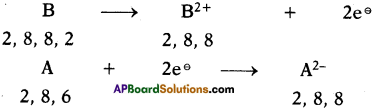iv) Which element will be oxidised in forming the ion?
B is losing electrons. So it is oxidised. .

v) When the elements react, what will be the nature of the bond formed?
When the elements react they form ionic bond because B is metal and A is non-metal.

Question 13.
Give one example for each of the following.
i) A polar covalent bond formed from two dissimilar atoms one of which is oxygen.
In the formation water molecule, polar covalent bond is formed between two dissimilar atoms hydrogen and oxygen.

ii) A non-polar covalent compound formed from two similar atoms.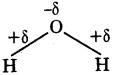The examples for a non-polar covalent compound formed from two similar atoms are Fluorine (F2), Chlorine (Cl2).

iii) A solid non-polar covalent compound.
The example for solid non-polar covalent compound is Iodine (I2).

iv) A polar covalent compound containing three shared pairs of electrons.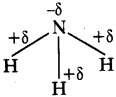The polar covalent compound containing three shared pairs of electrons is Ammonia.

Question 14.
The list of some substances is given below.
HCl, H2O, Cl2, NaBr, CH4, NH3, N2, O2, CaO, HF, I2 and Br2.
From the list above choose the substance / substances.

i) Which have only ionic bond in the molecules?
NaBr, CaO have only ionic bond in the molecules,

ii) Which has a triple covalent bond in its molecule?
N2 has a triple covalent bond in its molecule ($$\mathbf{N} \equiv \mathbf{N}$$).

iii) Which are solids?
Generally ionic compounds are solids. So NaBr, CaO are solids. I2 being a covalent compound still it is solid.

iv) Which has a double covalent bond in its molecule?
O2 has a double covalent bond in its molecule (O = O).

v) Which is non-polar covalent liquid?
The non-polar covalent liquid is Br2.Question 15.
There are elements with atomic numbers 4, 14, 8, 15 and 19. From this information answer the following questions.
a) A solid non-metal of valency 3.
The element with atomic number 15 is phosphorus. Its electronic configuration is 2, 8, 5. So its valency is 3 and it is a solid non-metal.

b) A gas of valency 2.
The element with atomic number 8 is oxygen. Its electronic configuration is 2, 6. So its valency is 2 and it is a gas.

c) A metal of valency 1.
The element with atomic number 19 is potassium. Its electronic configuration is 2, 8, 8, 1. So its valency is 1 and it is a metal.

d) A non metal of valency 4.
The element with atomic number 14 is silicon. Its electronic configuration is 2, 8, 4. Its valency is 4 and it is a non-metal.

Question 16.
Explain the following.
a) Ionic compounds conduct electricity.
Ionic compounds can be electrolysed to give their constituent ions. The ions obtained by dissociation move freely in solution and hence conduct electricity. When ionic compound is dissolved in water or melted, it becomes a good conductor of electricity.

b) Ionic compounds have high melting and boiling points while covalent compounds have low melting and boiling points.
The forces existing between ionic compounds are electrostatic in nature. They are strong forces. So in order to break these forces lot of energy is required. Therefore ionic compounds have higher boiling and melting points.

c) Ionic compounds dissolve in water whereas covalent compounds do not.
Ionic compounds are polar in nature. So they are soluble in polar solvent like water whereas covalent compounds are non-polar in nature. So they are insoluble in water.

d) Ionic compounds are usually hard crystals.
Due to strong attractive forces they are usually hard crystals.

Question 17.
If A, B, C and D are elements given that B is an inert gas (not Helium).

 Element Atomic Number A Z- 1 B Z C Z + 1 D Z + 4

What type of bonding would take place between (i) A and C and (ii) D and A and write their formulae?
1) Given that B is inert gas.
So the valency of A is 1 and it is a non-metal and valency of C is 1 and it is metal and valency of D is 4 and it is a non-metal.

2) The bond formed between C and A is ionic nature and its formula is CA.
The bond formed between D and A is covalent nature and its formula is DA4.

Question 18.
State whether the following statements are true or false. If statement is false, correct it.
i) In polar compounds the electropositive atom attracts the electron pair towards it.
This statement is wrong. In polar compounds the more electronegative atom attracts the electron pair towards it which is known as electronegativity.

ii) Hydrogen chloride gas is a di-polar molecule.
Chlorine is more electronegative than Hydrogen. So the electron pair shifts more towards chlorine atom. Thus partial positive charge is formed on Hydrogen and partial negative charge is formed on Chlorine. So it is dipolar molecule. So the statement is true.

iii) Covalent compounds are generally gases due to presence of weak electrostatic forces of attraction.
This statement is false. The forces are not electrostatic and also they are weak.

iv) Atoms achieve stable electronic configuration only by transfer of electrons from one atom to another.
This statement is false because they can acquire by not only electron transfer but also mutually sharing of electrons.

v) Ionic compounds are soft, solids or liquids at ordinary temperature.
This statement is false because ionic compounds are generally hard solids.

Question 19.
Five atoms are labelled from A to E.

 Atoms Atomic Number Mass Number A 20 40 B 9 19 C 3 7 D 8 16 E 7 14

a) Which one of these atoms (i) contain 7 protons, (ii) has an electronic configuration 2, 7?
i) The atom E contains 7 protons because its atomic number is 7.
ii) The atom with electronic configuration 2, 7 is B because its atomic number is 9.

b) Write down the formula of the compound formed between C and D.
The electronic configuration of C is 2, 1 since its atomic number is 3. The electronic configuration of D is 2, 6 since its atomic number is 8.
So the valency of C is 1. –
The valency of D is 2 (8 – 6 = 2).
∴ The compound formed between C and D is C2 D.

c) Predict which are (i) metals, (ii) non-metals?
Metals are A, C.
Non-metals are B, D, E.Question 20.
Can you suggest an experiment to prove that ionic compounds possess strong bonds when compared to that of covalent bonds? Explain the procedure.

• Take a small amount of sodium chloride (NaCl) on a metal spatula (having an insulated handle).
• Heat it directly over the flame of a burner.
• We will see that sodium chloride (NaCl) does not melt easily.
• Sodium chloride melts (and becomes a liquid) only on strong heating.
• This shows that sodium chloride which is an ionic compound possesses strong bonds.
• So it has a high melting point.
• Whereas covalent compound like naphthalene and carbon tetra chloride has low boiling points such as 80° C and 77° C respectively.
• The force of attraction between the molecules of a covalent compound is very weak.
• Only a small amount of heat energy is required to break these weak molecular forces, due to which covalent compound has low melting points and low boiling points.

Question 21.
The electronic configurations of following elements are given below.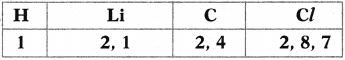From these values complete the table.

 Compound Type of bonding Lithium chloride Lithium hydride Hydrogen chloride Carbon tetrachloride

a) Lithium is metal and chlorine is non-metal. So the bond is ionic in nature.
b) Lithium is metal and hydrogen is non-metal. So the bond is ionic in nature.
c) Hydrogen and chlorine are two dissimilar non-metals. So the bond formed is polar covalent bond.
d) Carbon and chlorine are dissimilar non-metals but electronegativity difference is less. So they form non-polar covalent bond.

 Compound Type of bonding Lithium chloride Ionic bond Lithium hydride Ionic bond Hydrogen chloride Polar covalent bond Carbon tetrachloride Non-polar covalent bond

Question 22.
Draw the structure of O2 by using valence bond theory.
O2 Formation :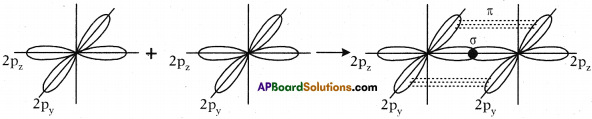Question 23.
Draw the structure of N2.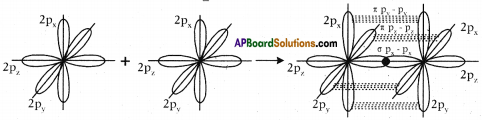Question 24.
Draw the structure of molecules by Lewis method.
Formation of F2: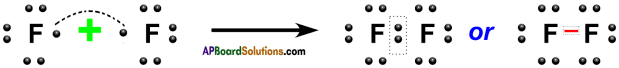Formation of O2 :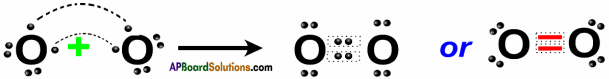Formation of N2 :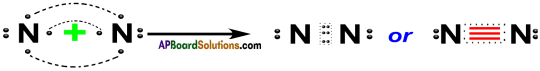Formation of CH4 :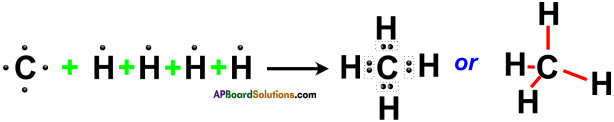Formation of NH3 :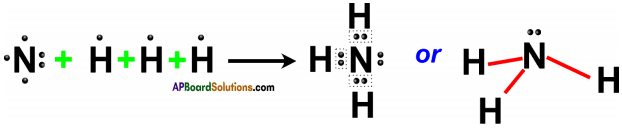Formation of H2O :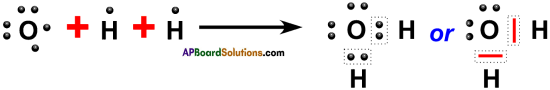Formation of BeCl2 :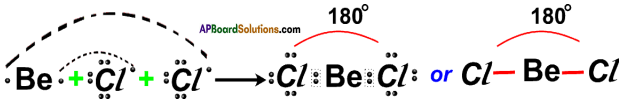Formation of BF3 :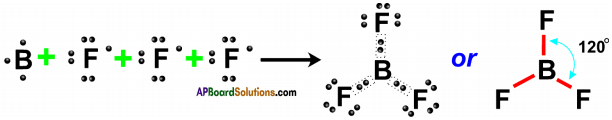Question 24.
Explain the formation of the following molecules using Lewis theory.
a) N2
b) O2
(OR)
Write the formation of double bond and triple bond according to Lewis theory.
a) Formation of N2 molecule by Lewis theory :

1. The electronic configuration of ’N’ atom is 2, 5 and to have octet in the valence shell it requires three more electrons.
2. When two nitrogen atoms approach each other, each atom contributes 3 electrons for bonding.
3. There are six electrons shared between two nitrogen atoms in the form of three pairs.
4. Therefore, there is a triple bond between two nitrogen atoms in N2 molecule.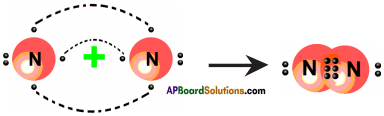b) Formation of O2 molecule by Lewis theory :

1. The electronic configuration of 8O is 2, 6.
2. Oxygen atom has six electrons in its valence shell.
3. It requires two more electrons to get octet in its valence shell.
4. Therefore oxygen atoms come close and each oxygen atom contributes two electrons for bonding.
5. Thus, there exist two covalent bonds between two oxygen atoms in O2 molecule as there are two pairs of electrons shared between them.
6. Two pairs of electrons are distributed between two oxygen atoms.
7. So, we can say that a double bond is formed between two oxygen atoms in O2 molecule.
8. By viewing the following diagram, both the oxygen atoms have octet in the valence shell.